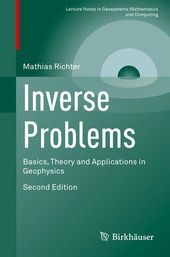# Inverse Problems

Basics, Theory and Applications in Geophysics

Birkhäuser (Verlag)
• 2. Auflage
• |
• erscheint ca. am 27. Januar 2021

• Buch
• |
• Softcover
• |
• X, 253 Seiten
978-3-030-59316-2 (ISBN)

This textbook is an introduction to the subject of inverse problems with an emphasis on practical solution methods and applications from geophysics. The treatment is mathematically rigorous, relying on calculus and linear algebra only; familiarity with more advanced mathematical theories like functional analysis is not required. Containing up-to-date methods, this book will provide readers with the tools necessary to compute regularized solutions of inverse problems. A variety of practical examples from geophysics are used to motivate the presentation of abstract mathematical ideas, thus assuring an accessible approach.
Beginning with four examples of inverse problems, the opening chapter establishes core concepts, such as formalizing these problems as equations in vector spaces and addressing the key issue of ill-posedness. Chapter Two then moves on to the discretization of inverse problems, which is a prerequisite for solving them on computers. Readers will be well-prepared for the final chapters that present regularized solutions of inverse problems in finite-dimensional spaces, with Chapter Three covering linear problems and Chapter Four studying nonlinear problems. Model problems reflecting scenarios of practical interest in the geosciences, such as inverse gravimetry and full waveform inversion, are fully worked out throughout the book. They are used as test cases to illustrate all single steps of solving inverse problems, up to numerical computations. Five appendices include the mathematical foundations needed to fully understand the material.
This second edition expands upon the first, particularly regarding its up-to-date treatment of nonlinear problems. Following the author's approach, readers will understand the relevant theory and methodology needed to pursue more complex applications. Inverse Problems is ideal for graduate students and researchers interested in geophysics and geosciences.

 Reihe: Auflage: 2nd ed. 2020 Sprache: Englisch Verlagsort: Cham | Schweiz Verlagsgruppe: Springer International Publishing Zielgruppe: Für Beruf und Forschung Editions-Typ: Überarbeitete Ausgabe Illustrationen: X, 253 p. Maße: Höhe: 23.5 cm | Breite: 15.5 cm Schlagworte: ISBN-13: 978-3-030-59316-2 (9783030593162) DOI: 10.1007/978-3-030-59317-9weitere Ausgaben werden ermittelt
Matthias Richter is Professor of Mathematics at Universität der Bundeswehr München, Faculty of Electrical Engineering and Information Technology. He has conducted industrial research with Siemens and Infineon Technologies for fourteen years.

Characterization of Inverse Problems.- Discretization of Inverse Problems.- Regularization of Linear Inverse Problems.- Regularization of Nonlinear Inverse Problems.- Appendix A. Results from Linear Algebra.- Appendix B. Function Spaces.- Appendix C. The Fourier Transform.- Appendix D. Regularization Property of CGNE.- Appendix E. Existence and Uniqueness Theorems for Waveform Inversion.

Schweitzer Klassifikation
• Geowissenschaften
•Geophysik
• Mathematik
•Algebra
• Mathematik
•Analysis
• Mathematik
•Angewandte Mathematik
•Numerische Mathematik
• Mathematik
•Zahlentheorie
Thema Klassifikation
Newbooks Subjects & Qualifier
• Geowissenschaften
•Geologie
•Geophysik
DNB DDC Sachgruppen
Dewey Decimal Classfication (DDC)
• Science
•Earth sciences & geology
•Earth sciences
• Science
•Mathematics
•Mathematics
• Science
•Mathematics
•Numerical analysis
• Science
•Physics
•Physics
BIC Classifikation
• Mathematics & science
•Mathematics
•Applied mathematics
•Mathematical modelling
• Mathematics & science
•Mathematics
•Calculus & mathematical analysis
•Numerical analysis
• Mathematics & science
•Physics
•Applied physics
•Geophysics
• Mathematics & science
•Physics
•Mathematical physics
BISAC Classifikation
• Mathematics
•Applied
• Mathematics
•Number Systems
• Science
•General
• Science
•Physics / General
•Mathematical & Computational
Warengruppensystematik 2.0

Noch nicht erschienen

 53,49 € inkl. 5% MwSt.